Listor / Teknisk fysik / Miniprojekt Newton’s Mill

# Miniprojekt Newton’s Mill

## Introduction

On a visit to England, we began to engage in a project concerning Newton's Mill. The old mill is filled with water to a height of 20 feet. Our first task in the project was to calculate the hydrostatic pressure that the water pushing on the walls using a Matlab program.

The pond needs renovation through strengthening the dam walls. Our second task of the project was that from a safety perspective calculate the new hydrostatic pressure when the dam gets its regular shape depending on the water level. The water level in the late summer is very low but in the spring it risesup to 100 feet.  Our third task was to write an algoritm that sets the length of the subintervalls automatically so that the result will calculated with a precison of four correct decimales.

The personal scope of the project is to gain a deeper understanding of the relevant topics in

the course Scientific Computing 1. The topics that will be treated are fundamental

Programming structures in Matlab, problem solving methodology and understanding of the solution of integrals by the methods traps and quad.

## Problemsolving

### Part 1

Our strategy here was to first store the given values of the water level and the dams width in vectors which we were given the task. Then we created a function that calculates the hydrostatic pressure by using the given parameters. The equations we received by the Manager:

w=40-20*exp(-(0.01*y).^2);

M=p.*(D(k)-y).*w;

The equations above means that the pond width w is exposed by a the pressure M that the dam exerts on the walls.

After this, a new vector s with five elements where we in the for-loop stores the calculated pressures. We chosed to use Matlabs trapz commando in which we are submitting vectors for height and pressure for the different heights. Since we in this task only had values in given points and no function of the press depending on the altitude, we used trapz instead of the INTEGRAL or quad.

I=trapz(y,s)

The result of the integral stored in the integral I as then was printed.

### Part 2

To solve this part we were given a function about how the width of the dam varies depending on what water level we measure.

w(y) = 40*20e^(0.01y)^2, w=width and y=water level.

Our task was to determine the hydrostatic pressure for each water level beteween 10 and 100 foot with a step length of 0,5 foot. The pressure were given by the integral: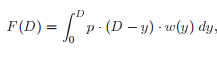D=The water level at a specific time, p=62:5 lb/ft^3

Since we got the information in terms of a function we decided to use Matlabs quad commando to calculte the integral. By using a for-loop we could integrate for each value of D.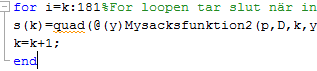### Part 3

To get a result with an accurancy that met given tolerance we wrote a while-loop that reapeted the calculations of the error until it was smaller than the tolerance. For each loop we reduced the length of the subintervall by its half. We aslo saved the correct length and the estimated error in a vector to make their values easier to plot.To make a calculation for each value that the water level could adopt, we implemented a for-loop in the beginning of the script.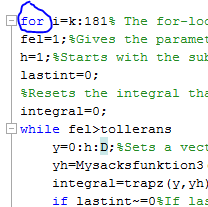When the calculations are finished we plot the results in three different graphs.

## Result

### Part 1 example

The hydrostatic pressure in bar is:

2.5181e+05

### Part 2 example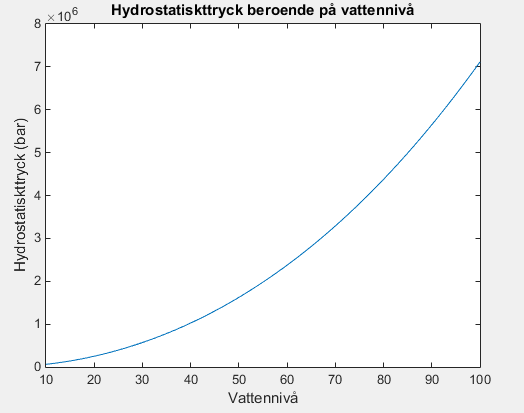Figure 1:The graph shows the hydrostatic pressure depending on the water level.

### Part 3 example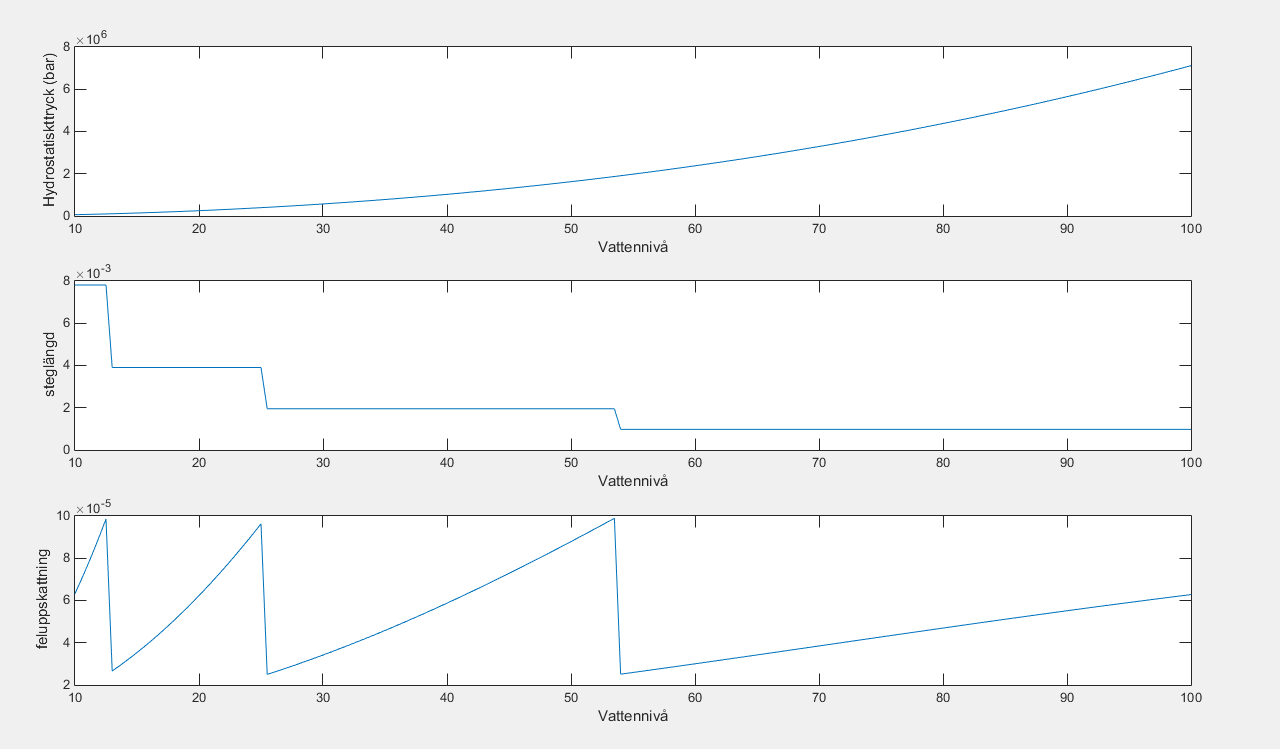Figure 2: Graph 1: Shows the hydrostatic pressure depending on the water level.

Graph 2: Shows the length of the subintervalls depending on the water level.

Graph 3: Shows the estimated error depending on the water level.

The result in part 3 shows us that length of the subintervall decreaces when the water level rises. The third graph, illustrating the estimated error, tells us for which water levels we should be extra cautious with. For example is the error big around a water level of 50 foot.

## Discussion

What we managed to achieve with this project is to share a calculated pressure using the given facts and Matlab function trapz. In part two we showed how the pressure changes depending on the water level. In part three, we wrote an algorithm that automatically gave us the discretization error for us, and the value of the step length. And at last we sketched three graphs of the results.

Some of the most important lessons we take with us from the project is how to build and implement an algorithm, what discretization is and how it can be applied and affects earnings. We have also got more knowledge about how functions are used. During our programming process we felt that we got some advantage from the lessons we had in class. The first and the second task was pretty easy to do when we knew how the Trapz- and Simpsons-method worked.

The hardest part of the assignment was, whitout any doubt,  to write an algorithm (as we did in task 3). When we read the instructions for the first time the problem seemed to be very complex. We tried to split the problem into pieces and focus at one piece at a time. We started with the piece that we thought was the most important and kept building from that. By solving the problem methodically part by part it became much easier.

## Appendix

### Part 1

w=[20.00 20.05 20.25 20.51 21.18];%Sets a vector with the measured values of the dams shape.

p=62.5;

y=0:5:20;%Sets a vector with the measured values of the water level.

s=zeros(1,5);

for i=1:5

s(i)=p*(20-y(i))*w(i); %The result from the measured values are saved in the vector s.

end

I=trapz(y,s); %The integral is solved with matlabs trapz commando.

disp('The hydrostatic pressure in bar: ');

disp(I);

### Part 2

p=62.5;

k=1;

D=10:0.5:100;%Sets a vector with all the values the water level can take.

s=zeros(1,181);%Empty vector with 181 elements.

for i=k:181%The for-loop ends when the integral has been calculated for all values the water level can take.

s(k)=quad(@(y)Mysacksfunktion2(p,D,k,y),0,D(k));%The results are saved in the vector s.

k=k+1;

end

plot(D,s);

title('The hydrostatic pressure depending on the water level');

xlabel('Water level (foot)');

ylabel('Hydrostatic pressure (bar)');

#### Function 2

function M=Mysacksfunktion2(p,D,k,y)

w=40-20*exp(-(0.01*y).^2);

M=p.*(D(k)-y).*w;

### Part 3

D=10;

losning=zeros(1,181);%Sets an empty vector where we will save the results later.  .

hvarden=zeros(1,181);%Empty vector where the length of the subintervalls will be saved.

Dintervall=10:0.5:100;%Empty vector with all the values the water level can take.

allafel=zeros(1,181);%Empty vector where the absolute errors will be saved.

tollerans=0.0001;

k=1;

for i=k:181% The for-loop ends when all values of the water level have been calculated.

fel=1;%Gives the parameter error a value so walk into the while-loop.

h=1;%Starts with the subintervall length 1.

lastint=0;

%Resets the integral that we are going to calculate.

integral=0;

while fel>tollerans

y=0:h:D;%Sets a vector with the subintervall length h from 0 to the current value of the water level.

yh=Mysacksfunktion3(62.5,D,y);%The solution is stored in yh.

integral=trapz(y,yh);%The integral is soleved with the matlab commando trapz.

if lastint~=0%If last inegral got a value( not equal to 0) the script walks into the foor-loop.

fel=abs(integral-lastint)/3;%The absolute error is calculated.

hvarden(i)=h;%The length of the subintervall is saved in element NO:i in the vector hvarden.

allafel(i)=fel;%The aboslute error is saved in element NO:i in the vector allafel.

end

lastint=integral;%Sets lastint=integral so the absolute error can be calculated in the next loop.

h=h/2;%The length of the subintervall is decreased.

end

losning(i)=integral;%When the while loop is done the variabel integral contains a result with an accurancy meets our tolerance.

D=D+0.5;

end

subplot(3,1,1);%three graphs are going to be plotted.

plot(Dintervall,losning);%Shows the hydrostatic pressure depending on the water level.

xlabel('Water level (foot)')

ylabel('Hydrostatic pressure (bar)')

subplot(3,1,2);

plot(Dintervall,hvarden);%Shows how the length of the subintervall changes when the water level varies.

xlabel('Water level (foot)')

ylabel('Subintervall length')

subplot(3,1,3);

plot(Dintervall,allafel);%Shows how the absolute error changes when the water level varies.

xlabel('Water level (foot)')

ylabel('Estimatedd error')

#### Function 3

function Mu=Mysacksfunktion3(p,D,y)

w=40-20*exp(-(0.01*y).^2);

Mu=p.*(D-y).*w;

Publiceringsdatum: 2014-11-26